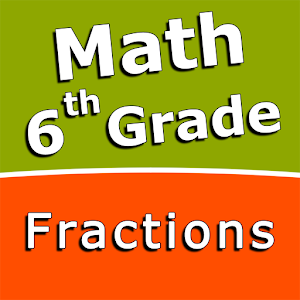Türkçe

Her gün ücretsiz lisanslı Android uygulamaları ve oyunlar sunuyoruz.\$2.99
SÜRESI DOLMUŞ

# Android Giveaway of the Day - Fractions and mixed numbers - 6th grade math

Add fractions and mixed numbers with like denominators.
\$2.99 SÜRESI DOLMUŞ
Kullanıcı puanı: 0 (0%) 0 (0%)

Bu programın süresi doldu. Fractions and mixed numbers - 6th grade math artık firmadan temin edilebilir.

Intuitive interface powered by handwriting input and a choice of three fun and engaging mini games in addition to a regular math trainer mode make our app stand out from the crowd of generic math learning apps.

With Fractions and mixed numbers - 6th grade math you can practice and improve the following math skills:
- Write fractions in lowest terms
- Add fractions and mixed numbers with like denominators
- Add fractions and mixed numbers with unlike denominators
- Subtract fractions and mixed numbers with like denominators
- Subtract fractions and mixed numbers with unlike denominators
- Multiply fractions and mixed numbers
- Multiply mixed numbers and whole numbers
- Divide fractions and mixed numbers
- Divide mixed numbers by whole numbers
- Convert fractions and mixed numbers to decimals

Sergey Malugin

Educational

### Sürüm:

Varies with device

### Boyut:

Varies with device

Everyone

### Uyumluluk:

Varies with device

## Fractions and mixed numbers - 6th grade math üzerine yorumlar

Thank you for voting!